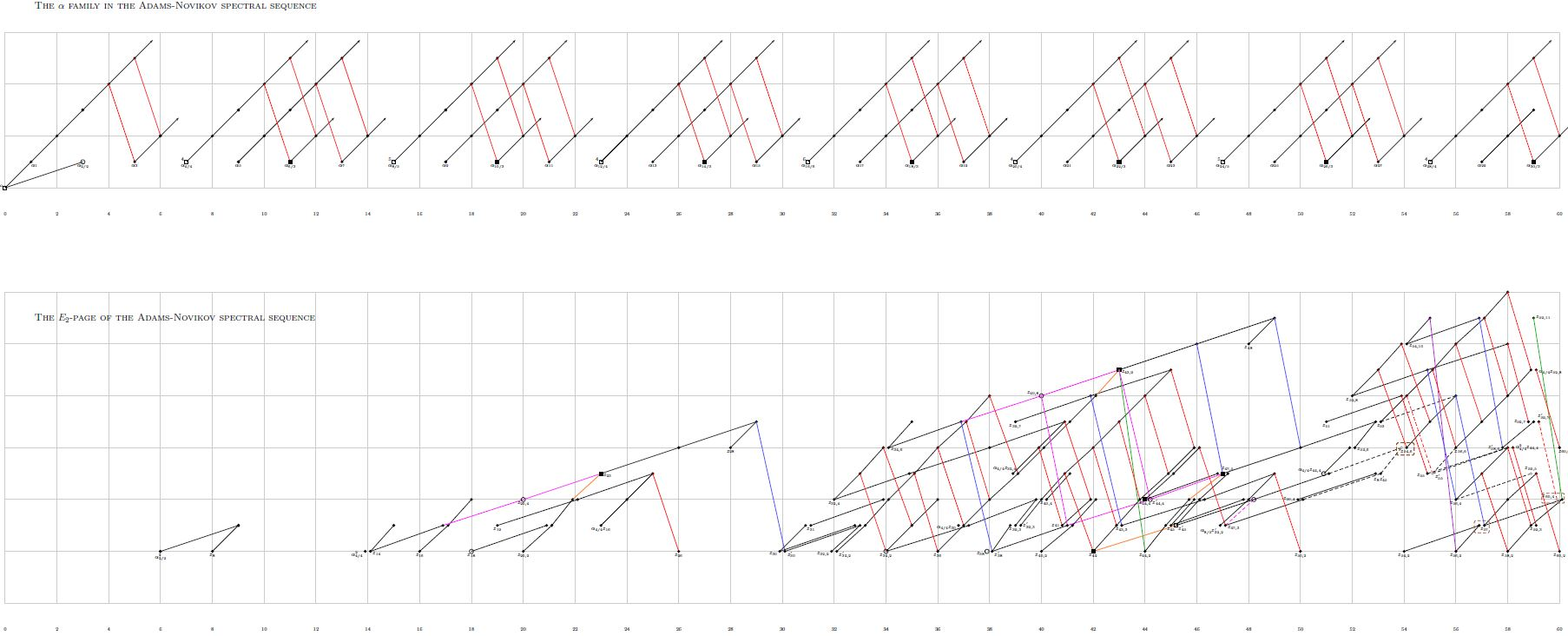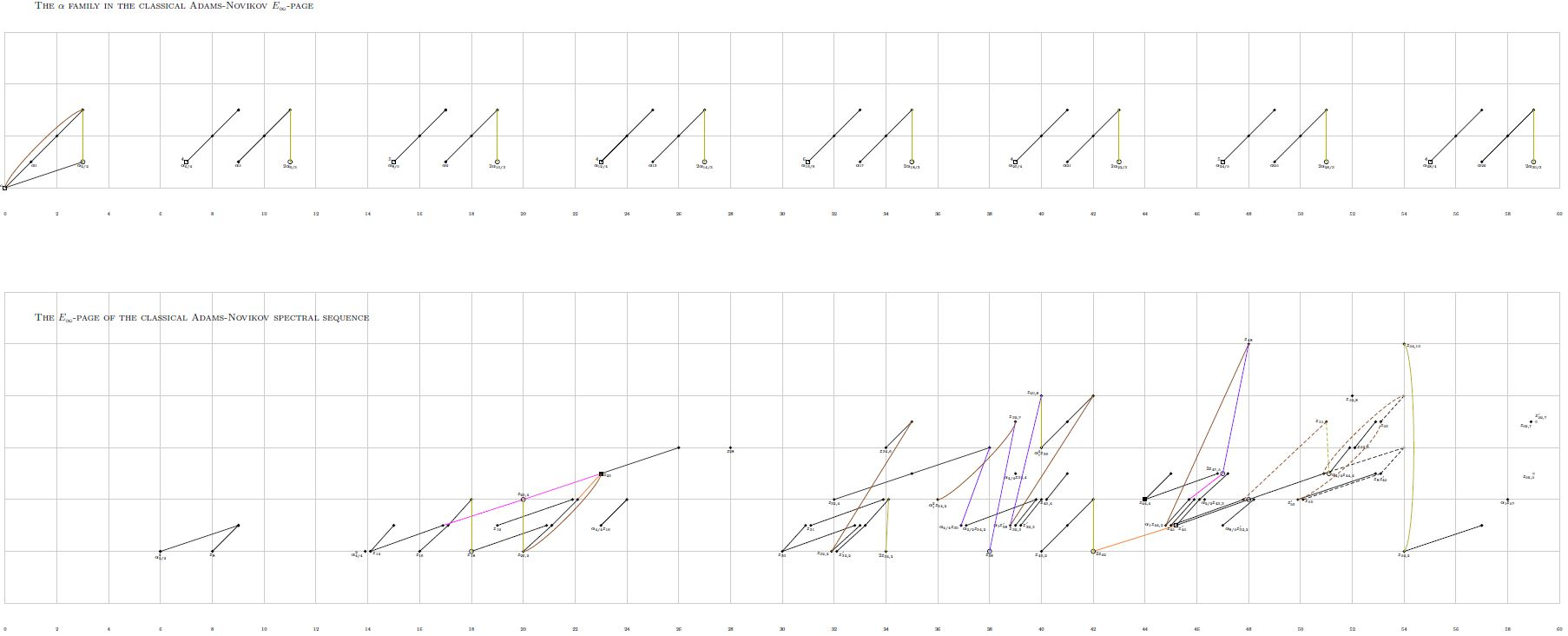Contents

### Context

#### Stable Homotopy theory

stable homotopy theory

Introduction

# Contents

## Idea

The Adams-Novikov spectral sequence is the $E$-Adams spectral sequence, for $E =$ MU.

More in detail, the Adams-Novikov spectral sequence is a class of spectral sequences, which refine the classical Adams spectral sequence by replacing ordinary cohomology with coefficients in Fp by complex cobordism cohomology theory MU. This makes the Adams-Novikov spectral sequence converge to the full homotopy groups of connective spectra, hence in particular to the full stable homotopy groups of spheres (Novikov 67, Theorems 3.1, 3.3, reviewed as Ravenel 78, Thm. 3.1).

More generally for $E$ any suitable E-infinity ring there is an Adams-Novikov-type spectral sequence involving $E$-generalized cohomology/generalized homology. This fully general notion is often again just referred to as the $E$-Adams spectral sequence. Accordingly, see there for more. For detailed introduction, see at Introduction to the Adams Spectral Sequence.

## Properties### Relation to Brown-Peterson spectrum

The $p$-component of the $E^2$-term of the Adams-Novikov spectral sequence for the sphere spectrum, hence the one converging to the stable homotopy groups of spheres $\pi_\ast(\mathbb{S})$ is

$Ext_{BP_\ast(BP)}(BP_\ast, BP_\ast) \,,$

where $BP$ denotes the Brown-Peterson spectrum at prime $p$.

recalled e.g. as Ravenel, theorem 1.4.2

The generalization of the Adams spectral sequence from $E =$ HA to $E =$ MU is due to

• Sergei Novikov, The methods of algebraic topology from the viewpoint of cobordism theories, Izv. Akad. Nauk. SSSR. Ser. Mat. 31 (1967), 855–951 (Russian) (Novikov67)

While that article was still being translated to English, Frank Adams learned of and then lectured about this work in 1967 in Chicago. This lecture together with two later lectures in 1970 and in 1971 constitute the book

Further early development of this Adams-Novikov spectral sequence:

• Raphael Zahler, The Adams-Novikov Spectral Sequence for the Spheres, Annals of Mathematics Second Series, Vol. 96, No. 3 (Nov., 1972), pp. 480-504 (doi:10.2307/1970821)

Review:

The Adams chart of the ANSS for large order has been presented in